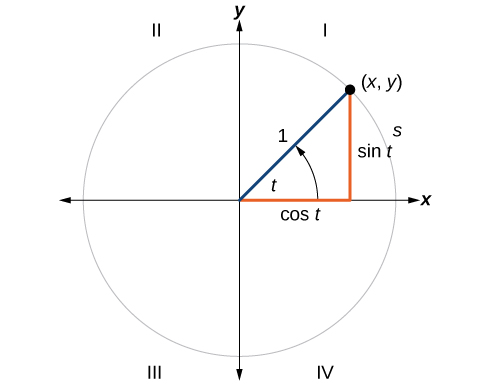# 7.3 Unit circle

 Page 1 / 11
In this section you will:
• Find function values for the sine and cosine of and
• Identify the domain and range of sine and cosine functions.
• Find reference angles.
• Use reference angles to evaluate trigonometric functions.The Singapore Flyer is the world’s tallest Ferris wheel. (credit: ʺVibin JKʺ/Flickr)

Looking for a thrill? Then consider a ride on the Singapore Flyer, the world’s tallest Ferris wheel. Located in Singapore, the Ferris wheel soars to a height of 541 feet—a little more than a tenth of a mile! Described as an observation wheel, riders enjoy spectacular views as they travel from the ground to the peak and down again in a repeating pattern. In this section, we will examine this type of revolving motion around a circle. To do so, we need to define the type of circle first, and then place that circle on a coordinate system. Then we can discuss circular motion in terms of the coordinate pairs.

## Finding trigonometric functions using the unit circle

We have already defined the trigonometric functions in terms of right triangles. In this section, we will redefine them in terms of the unit circle. Recall this a unit circle is a circle centered at the origin with radius 1, as shown in [link] . The angle (in radians) that $\text{\hspace{0.17em}}t\text{\hspace{0.17em}}$ intercepts forms an arc of length $\text{\hspace{0.17em}}s.\text{\hspace{0.17em}}$ Using the formula $\text{\hspace{0.17em}}s=rt,$ and knowing that $\text{\hspace{0.17em}}r=1,$ we see that for a unit circle, $\text{\hspace{0.17em}}s=t.$

The x- and y- axes divide the coordinate plane into four quarters called quadrants. We label these quadrants to mimic the direction a positive angle would sweep. The four quadrants are labeled I, II, III, and IV.

For any angle $\text{\hspace{0.17em}}t,$ we can label the intersection of the terminal side and the unit circle as by its coordinates, $\text{\hspace{0.17em}}\left(x,y\right).\text{\hspace{0.17em}}$ The coordinates $\text{\hspace{0.17em}}x\text{\hspace{0.17em}}$ and $\text{\hspace{0.17em}}y\text{\hspace{0.17em}}$ will be the outputs of the trigonometric functions $\text{\hspace{0.17em}}f\left(t\right)=\mathrm{cos}\text{\hspace{0.17em}}t\text{\hspace{0.17em}}$ and $\text{\hspace{0.17em}}f\left(t\right)=\mathrm{sin}\text{\hspace{0.17em}}t,$ respectively. This means andUnit circle where the central angle is   t   radians

## Unit circle

A unit circle    has a center at $\text{\hspace{0.17em}}\left(0,0\right)\text{\hspace{0.17em}}$ and radius $\text{\hspace{0.17em}}1.\text{\hspace{0.17em}}$ In a unit circle, the length of the intercepted arc is equal to the radian measure of the central angle $\text{\hspace{0.17em}}t.$

Let $\text{\hspace{0.17em}}\left(x,y\right)\text{\hspace{0.17em}}$ be the endpoint on the unit circle of an arc of arc length     $\text{\hspace{0.17em}}s.\text{\hspace{0.17em}}$ The $\text{\hspace{0.17em}}\left(x,y\right)\text{\hspace{0.17em}}$ coordinates of this point can be described as functions of the angle.

## Defining sine and cosine functions from the unit circle

The sine function relates a real number $\text{\hspace{0.17em}}t\text{\hspace{0.17em}}$ to the y -coordinate of the point where the corresponding angle intercepts the unit circle. More precisely, the sine of an angle $\text{\hspace{0.17em}}t\text{\hspace{0.17em}}$ equals the y -value of the endpoint on the unit circle of an arc of length $\text{\hspace{0.17em}}t.\text{\hspace{0.17em}}$ In [link] , the sine is equal to $\text{\hspace{0.17em}}y.\text{\hspace{0.17em}}$ Like all functions, the sine function    has an input and an output. Its input is the measure of the angle; its output is the y -coordinate of the corresponding point on the unit circle.

The cosine function    of an angle $\text{\hspace{0.17em}}t\text{\hspace{0.17em}}$ equals the x -value of the endpoint on the unit circle of an arc of length $\text{\hspace{0.17em}}t.\text{\hspace{0.17em}}$ In [link] , the cosine is equal to $\text{\hspace{0.17em}}x.$

Because it is understood that sine and cosine are functions, we do not always need to write them with parentheses: $\text{\hspace{0.17em}}\mathrm{sin}\text{\hspace{0.17em}}t\text{\hspace{0.17em}}$ is the same as $\text{\hspace{0.17em}}\mathrm{sin}\left(t\right)\text{\hspace{0.17em}}$ and $\text{\hspace{0.17em}}\mathrm{cos}t\text{\hspace{0.17em}}$ is the same as $\text{\hspace{0.17em}}\mathrm{cos}\left(t\right).\text{\hspace{0.17em}}$ Likewise, $\text{\hspace{0.17em}}{\mathrm{cos}}^{2}t\text{\hspace{0.17em}}$ is a commonly used shorthand notation for $\text{\hspace{0.17em}}{\left(\mathrm{cos}\left(t\right)\right)}^{2}.\text{\hspace{0.17em}}$ Be aware that many calculators and computers do not recognize the shorthand notation. When in doubt, use the extra parentheses when entering calculations into a calculator or computer.

#### Questions & Answers

write down the polynomial function with root 1/3,2,-3 with solution
if A and B are subspaces of V prove that (A+B)/B=A/(A-B)
write down the value of each of the following in surd form a)cos(-65°) b)sin(-180°)c)tan(225°)d)tan(135°)
Prove that (sinA/1-cosA - 1-cosA/sinA) (cosA/1-sinA - 1-sinA/cosA) = 4
what is the answer to dividing negative index
In a triangle ABC prove that. (b+c)cosA+(c+a)cosB+(a+b)cisC=a+b+c.
give me the waec 2019 questions
the polar co-ordinate of the point (-1, -1)
prove the identites sin x ( 1+ tan x )+ cos x ( 1+ cot x )= sec x + cosec x
tanh`(x-iy) =A+iB, find A and B
B=Ai-itan(hx-hiy)
Rukmini
what is the addition of 101011 with 101010
If those numbers are binary, it's 1010101. If they are base 10, it's 202021.
Jack
extra power 4 minus 5 x cube + 7 x square minus 5 x + 1 equal to zero
the gradient function of a curve is 2x+4 and the curve passes through point (1,4) find the equation of the curve
1+cos²A/cos²A=2cosec²A-1
test for convergence the series 1+x/2+2!/9x3ByByBy Nick SwainByByBy RhodesBy